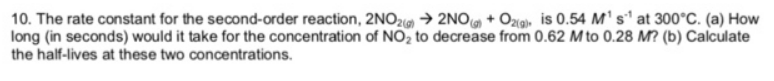# Problem: The rate constant for the second-order reaction, 2NO2(g) → 2NO(g) + O2(g), is 0.54 M-1 s-1 at 300°C. (a) How long (in seconds) would it take for the concentration of NO2 to decrease from 0.62 M to 0.28 M? (b) Calculate the half-lives at these two concentrations.

###### FREE Expert Solution
79% (145 ratings)###### Problem Details

The rate constant for the second-order reaction, 2NO2(g) → 2NO(g) + O2(g), is 0.54 M-1 s-1 at 300°C.

(a) How long (in seconds) would it take for the concentration of NO2 to decrease from 0.62 M to 0.28 M?

(b) Calculate the half-lives at these two concentrations.